florida holiday home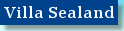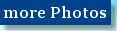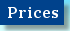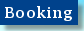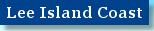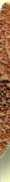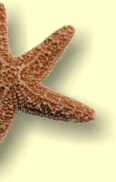### BookingWe would be very happy to welcome you to Villa Sealand!

Please look at the time-table below to check the availability.

2010

Jan
Feb
Mar
Apr
May
Jun
Jul
Aug
Sep
Oct Nov
Dec

1
H
M
M
M
M
O
O
O
O
M
M
M
Off Season
2
H
M
M
M
M
O
O
O
O
M
M
M
Main Season
3
H
M
M
M
M
O
O
O
O
M
M
M
Holidays
4
H
M
M
M
M
O
O
O
O
M
M
M
booked
5
M
M
M
M
M
O
O
O
O
M
M
M

6
M
M
M
M
M
O
O
O
O
M
M
M

7
M
M
M
M
M
O
O
O
O
M
M
M

8
M
M
M
M
M
O
O
O
O
M
M
M

9
M
M
M
M
M
O
O
O
O
M
M
M

10
M
M
M
M
M
O
O
O
O
M
M
M

11
M
M
M
M
M
O
O
O
O
M
M
M

12
M
M
M
M
M
O
O
O
O
M
M
M

13
M
M
M
M
M
O
O
O
O
M
M
M

14
M
M
M
M
M
O
O
O
O
M
M
M

15
M
M
M
M
M
O
O
O
O
M
M
M

16
M
M
M
M
M
O
O
O
O
M
M
M

17
M
M
M
M
M
O
O
O
O
M
M
M

18
M
M
M
M
M
O
O
O
O
M
M
M

19
M
M
M
M
M
O
O
O
O
M
M
M

20
M
M
M
M
M
O
O
O
O
M
M
H

21
M
M
M
M
M
O
O
O
O
M
M
H

22
M
M
M
M
M
O
O
O
O
M
M
H

23
M
M
M
M
M
O
O
O
O
M
M
H

24
M
M
M
M
M
O
O
O
O
M
M
H

25
M
M
M
M
M
O
O
O
O
M
M
H

26
M
M
M
M
M
O
O
O
O
M
M
H

27
M
M
M
M
M
O
O
O
O
M
M
H

28
M
M
M
M
M
O
O
O
O
M
M
H

29
M
M
M
M
M
O
O
O
O
M
M
H

30
M

M
M
M
O
O
O
O
M
M
H

31
M

M

M

O
O

M

H### BookingTo book accommodation in Villa Sealand please write a brief note to the following E-mail address:

info@florida-holiday-home.com